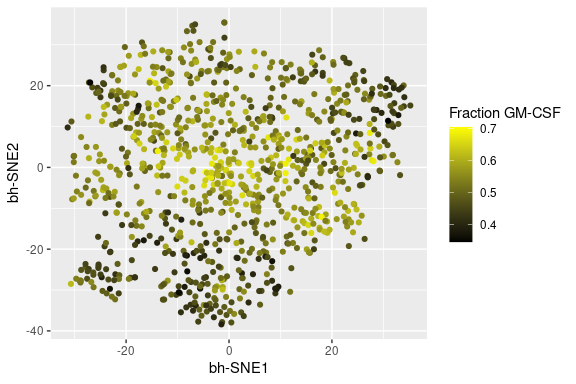# Assessing quality of CyTOF data with KNN

### CyTOF data quality

CyTOF data can potentially vary across replicates if there are problems with the process of sample preparation and/or data acquisition, among other things. Given that these samples are typically complicated, multi-subset specimens, measuing per-sample variance can be challenging. Here, we use our KNN to approximate the manifold overlap of two tubes. This can potentially be performed when variance is biologically driven as well. For the purpose of this vignette, we focus on data where the hypothesis is that there will be no variation between manifolds (and therefore all variation observed will be technical variation).

### KNN for differential abundance

There are many ways to assess differential abundance in CyTOF data (and high dimensional data in general). Here, we use our KNN as a proxy for variance across each cell in the manifold. In our case, we test two tubes that should in theory be the same (eg. PBMCs from the same donor, with the same markers). SCONE automatically outputs the per-knn percentage of cells in the non-baseline condition, shown below. The dataset we use is the Wanderlust dataset (paper: https://www.ncbi.nlm.nih.gov/pubmed/24766814, dataset: https://www.c2b2.columbia.edu/danapeerlab/html/wanderlust-data.html), between a single normal and IL-7 treated patient.

library(Sconify)
wand.final$IL7.fraction.cond.2[1:10] ##  0.6000000 0.4000000 0.7000000 0.5000000 0.7666667 0.2666667 0.3333333 ##  0.4666667 0.4666667 0.5000000 knitr::opts_chunk$set(fig.width=6, fig.height=4) 

We can plot this as a distrubtion between 0 and 100%. We hypothesize that this distribution of n cells and k nearest neighbors per cell will have the median and standard deviation of a coin tossed k times, with this action repeated n times. This is known formally as the binomial distribution with a probability set at 0.5. The analysis is below.

# Visualization
MakeHist(wand.final, 30, "IL7.fraction.cond.2", "fraction IL7")
## Warning: Use of dat[[grep(column.label, colnames(dat))]] is discouraged. Use
## .data[[grep(column.label, colnames(dat))]] instead.
## Warning: Removed 2 rows containing missing values (geom_bar).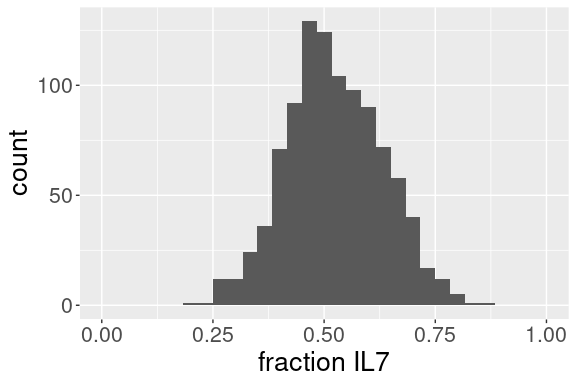# Characteristics of the visualization
median(wand.final$IL7.fraction.cond.2) ##  0.5 sd(wand.final$IL7.fraction.cond.2)
##  0.1083417

We now compare to our coin flipping.

# Compare to a coin toss distribution
# Flips a coin k number of times, n repetitions, and returns the vector
# containing the per-k percent heads
KFlip <- function(k, n) {
# The result
result <- sapply(1:n, function(i) {
# Get the fraction heads for n flips
flips <- sample(c(0, 1), k, replace = TRUE)
})
}

coin.toss <- data.frame("binomial" = KFlip(30, 1000))
MakeHist(coin.toss, 30, "binomial", "fraction heads")
## Warning: Use of dat[[grep(column.label, colnames(dat))]] is discouraged. Use
## .data[[grep(column.label, colnames(dat))]] instead.
## Warning: Removed 2 rows containing missing values (geom_bar).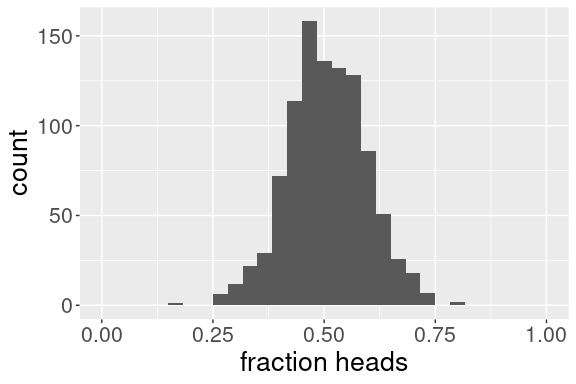median(coin.toss$binomial) ##  0.5 sd(coin.toss$binomial)
##  0.08885004

We can see that the Wanderlust dataset example has a standard deviation that is higher (0.08) than that of the aforementioned binomial distribution (0.05). We can also see that the Wanderlust dataset distribution has a slight tail to the right. If we consider the Wanderlust dataset as a “benchmark” in terms of data quality, we can see that new CyTOF datasets should be within a few hundreths of the standard deviation of the binomial distribution with the same number of trials as the number of nearest neighbors used.

Of note, we can approximate the standard deviation of the binomical distribution with a probability of 0.5 for any value k by using the formula sd = 0.5/sqrt(k). This is derived from the DeMoivre-Laplace theorem https://en.wikipedia.org/wiki/De_Moivre%E2%80%93Laplace_theorem. This means one does not need to simulate a distibution of coin tosses every time the k in the KNN is changed. I do it here just to show what it looks like.

Given that the observed variation (sd = 0.108) is higher than that of our aforementioned binomial distribution (sd = 0.093), and we observed the slight tail to the right, we hypothesize that this slight tail to the right could be a specific cell subset that is overrepresented in the IL-7 tube. To this end, we visualize our results colored on a t-SNE map below. If our hypothesis is true, a specific region of the map will be enriched by higher “fraction IL-7” values.

# Raw per-cell IgM levels
TsneVis(wand.final, "IgM(Eu153)Di", "Raw IgM expression")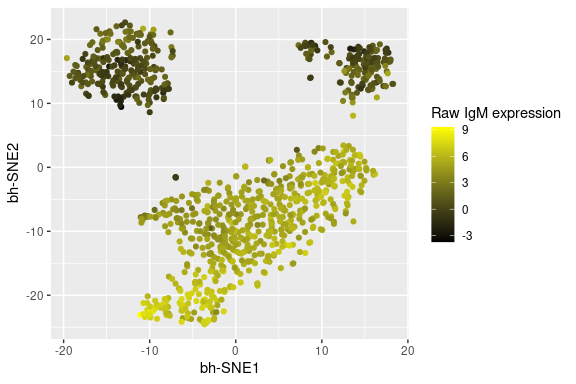TsneVis(wand.final, "IL7.fraction.cond.2", "Fraction cells in Il7 condition")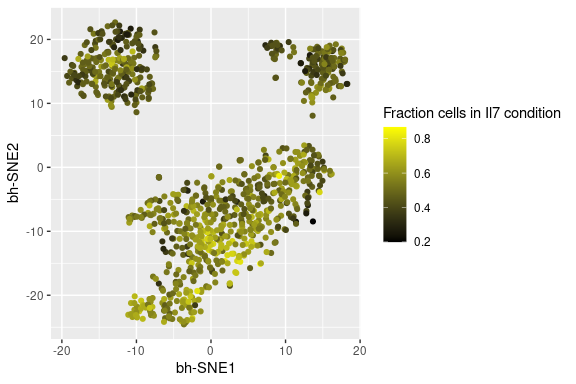One can see with the t-SNE map that the IL-7 condition appears to be slightly overrepresented in the more mature B cell compartment, as represented above by high IgM expression, which is consistent with our hypothesis. Given that the IL-7 stimulatory condition was only for 15 minutes (and therefore likely did not kill the stem cells), the result here leads to the hypothesis that the IL-7 tube had slightly more mature B cells in it than stem cells to begin with. While this particular case is not substantial and did not affect the identification of the IL-7 responsive population, results like this could help a user fine-tune a data analysis pipeline using this hypothesis-driven search for technical artifact.

### The case for normalization

The following is an example where normalization would be appropriate. I provide per-marker quantile normalization and z score transformation as options, but there are a number of normalization functions that could be performed on one’s data, after it is matrix form using fcs.to.tibble or process.multiple.files. I introduce untreated and treated data from the initial Nolan Lab barcoding paper (https://www.ncbi.nlm.nih.gov/pubmed/22902532). This dataset was produced when CyTOF was a very new technology, and therefore the data quality is considerably less than what one would observe today.

Below is the concatenated data of untreated cells and those treated with GM-CSF. We ran this analysis on 10,000 cells with a k of 100, but for this package we subsampled to 1000 post-analysis. This is a 15 minute stimulation, which means that any variation in the manifold between conditions is likely technical (though there are exceptions depending on the stiulation and marker). Recall that the best overlap would resemble aforementioned binomial distribution. Thus, we see substantial technical variation. The histogram below it is the same dataset, but we have performed quantile normalization and z-score transformation prior to the KNN computation. Notice that this histogram is substantially tighter, with a standard deviation of 0.06, comparable to our binomial distribution’s standard deviation of 0.05 if the k is 100 (0.5/sqrt(100)).

# Before normalization
MakeHist(bz.gmcsf.final, 100, "fraction.cond2", "fraction GM-CSF")
## Warning: Use of dat[[grep(column.label, colnames(dat))]] is discouraged. Use
## .data[[grep(column.label, colnames(dat))]] instead.
## Warning: Removed 2 rows containing missing values (geom_bar).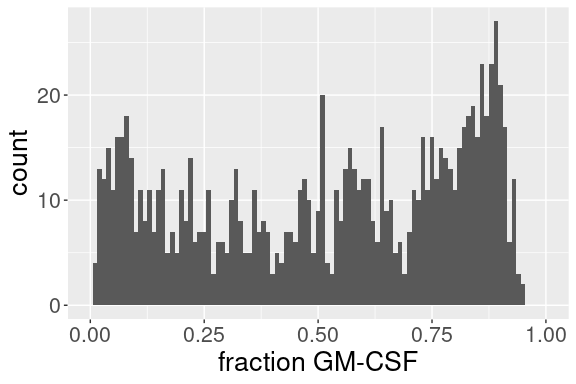# Characteristics of the visualization
median(bz.gmcsf.final$fraction.cond2) ##  0.5675 sd(bz.gmcsf.final$fraction.cond2)
##  0.2921823
# After normalization
MakeHist(bz.gmcsf.final.norm.scale, 100, "fraction.cond2", "fraction GM-CSF")
## Warning: Use of dat[[grep(column.label, colnames(dat))]] is discouraged. Use .data[[grep(column.label, colnames(dat))]] instead.
## Removed 2 rows containing missing values (geom_bar).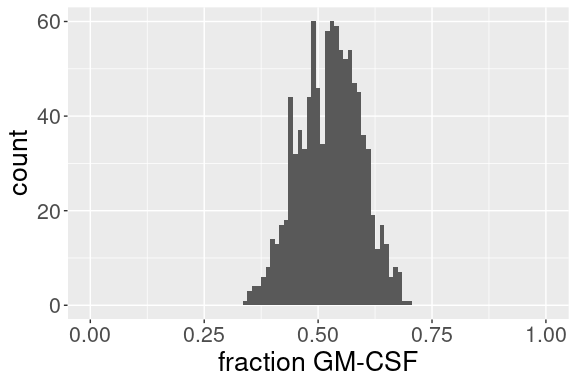# Characteristics of the visualization
median(bz.gmcsf.final.norm.scale$fraction.cond2) ##  0.53 sd(bz.gmcsf.final.norm.scale$fraction.cond2)
##  0.06722158

The above data suggests that normalization and z-score transformation can improve the quality of a dataset, as measured here by manifold overlap of markers not expected to change.

Below, we show t-SNE maps to determine if there are specific regions of the manifold where these changes are occurring. Note that the t-SNE maps will have a different shape, becasue these are two separate runs.

We observe that non-normalized data appears to have a global “drift” in the northeast direction of the map. The data suggests that the shift we observe between the untreated and treated manifold is not subset-specific. This dataset as acquired on a CyTOF 1 Mass Cytometer prior to bead-based normalization (https://www.ncbi.nlm.nih.gov/pubmed/23512433), so we hypothesize that this global drift could be the result of the mass cytometer acquisition performance changes occurring while the data were being acquired. If this is true, then this will luckily not be a problem for modern users. Nonetheless, our KNN-based manifold overlap analysis will be able to detect and quantify differences in high dimensional space, and can be used as an evaluation metric for technical (eg. barcoding) and analytical (eg. z-score transformation) improvements.

# t-SNE map colored by KNN-based fraction GM-CSF
TsneVis(bz.gmcsf.final, "fraction.cond2", "Fraction GM-CSF")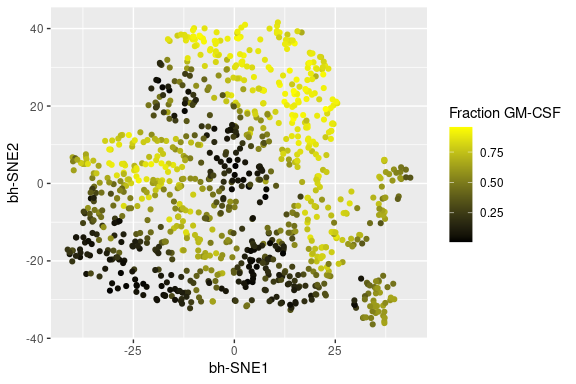# t-SNE map colored by KNN-based fraction GM-CSF
TsneVis(bz.gmcsf.final.norm.scale, "fraction.cond2", "Fraction GM-CSF")